# JP3739550B2 - Method for determining wafer measurement position - Google Patents

## Info

Publication number
JP3739550B2
JP3739550B2 JP31428697A JP31428697A JP3739550B2 JP 3739550 B2 JP3739550 B2 JP 3739550B2 JP 31428697 A JP31428697 A JP 31428697A JP 31428697 A JP31428697 A JP 31428697A JP 3739550 B2 JP3739550 B2 JP 3739550B2
Authority
JP
Japan
Prior art keywords
wafer
image
measurement
position
stage
Prior art date
Legal status (The legal status is an assumption and is not a legal conclusion. Google has not performed a legal analysis and makes no representation as to the accuracy of the status listed.)
Expired - Fee Related
Application number
JP31428697A
Other languages
Japanese (ja)
Other versions
JPH11135601A (en
Inventor

Original Assignee

Priority date (The priority date is an assumption and is not a legal conclusion. Google has not performed a legal analysis and makes no representation as to the accuracy of the date listed.)
Filing date
Publication date
Application filed by 大日本スクリーン製造株式会社 filed Critical 大日本スクリーン製造株式会社
Priority to JP31428697A priority Critical patent/JP3739550B2/en
Priority claimed from KR1019980035173A external-priority patent/KR100292936B1/en
Publication of JPH11135601A publication Critical patent/JPH11135601A/en
Application granted granted Critical
Publication of JP3739550B2 publication Critical patent/JP3739550B2/en
Anticipated expiration legal-status Critical
Application status is Expired - Fee Related legal-status Critical

## Images

•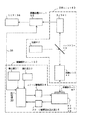•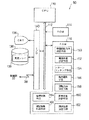•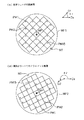•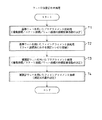•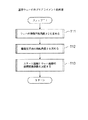•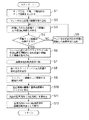•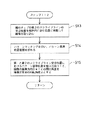••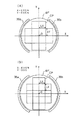••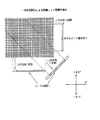•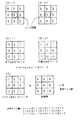•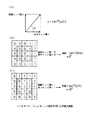•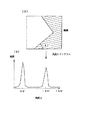•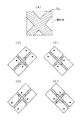•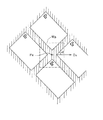•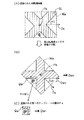•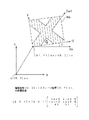•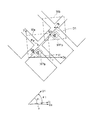•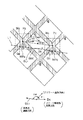•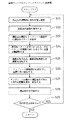•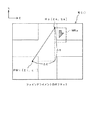•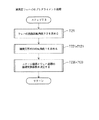•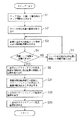•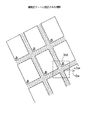••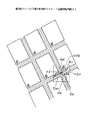•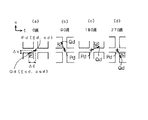•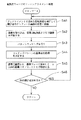•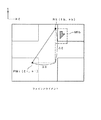## Classifications

• GPHYSICS
• G06COMPUTING; CALCULATING; COUNTING
• G06KRECOGNITION OF DATA; PRESENTATION OF DATA; RECORD CARRIERS; HANDLING RECORD CARRIERS
• G06K9/00Methods or arrangements for reading or recognising printed or written characters or for recognising patterns, e.g. fingerprints
• G06K9/20Image acquisition
• G06K9/32Aligning or centering of the image pick-up or image-field
• G06K9/3208Orientation detection or correction, e.g. rotation of multiples of 90 degrees
• GPHYSICS
• G06COMPUTING; CALCULATING; COUNTING
• G06TIMAGE DATA PROCESSING OR GENERATION, IN GENERAL
• G06T7/00Image analysis
• G06T7/70Determining position or orientation of objects or cameras
• G06T7/73Determining position or orientation of objects or cameras using feature-based methods
• G06T7/74Determining position or orientation of objects or cameras using feature-based methods involving reference images or patches
• GPHYSICS
• G06COMPUTING; CALCULATING; COUNTING
• G06TIMAGE DATA PROCESSING OR GENERATION, IN GENERAL
• G06T2207/00Indexing scheme for image analysis or image enhancement
• G06T2207/30Subject of image; Context of image processing
• G06T2207/30108Industrial image inspection
• G06T2207/30148Semiconductor; IC; Wafer

## Description


BACKGROUND OF THE INVENTION
The present invention relates to a technique for creating measurement information relating to a measurement point on a semiconductor wafer and determining the position of the measurement point using this measurement information.

[Prior art]
A semiconductor wafer is measured by various measuring devices in its manufacturing process. In the measurement process, a “positioning process (alignment process)” for accurately positioning a measurement probe (such as an optical element or an electrode) at a predetermined measurement point on the wafer is performed.

[Problems to be solved by the invention]
By the way, there are cases where it is desired to perform the same measurement using a plurality of measuring devices. However, when a wafer is set in a plurality of measuring apparatuses, the orientation and position of the wafer differ among the respective apparatuses, so that the coordinates of the measurement points on the wafer also take different values. Therefore, conventionally, there has been a problem that measurement information including a measurement position cannot be diverted between a plurality of measurement apparatuses.

Even when the same measuring apparatus is used, when a plurality of wafers are mounted, the coordinates of the measurement points on each wafer may take different values. For example, when the orientation of the wafer set in the measurement apparatus is not always exactly constant and can take an arbitrary angle to some extent, the coordinates of the measurement point take different values for each wafer. Therefore, there is a problem that it is difficult to accurately determine the position of the measurement point even in the same measurement apparatus.

The present invention has been made to solve the above-described problems in the prior art, and a first object of the invention is to provide a technique capable of diverting measurement information including measurement positions between a plurality of measurement apparatuses. And A second object is to provide a technique capable of determining the position of a measurement point on a wafer using such measurement information.

[Means for solving the problems and their functions and effects]
In order to solve at least a part of the above-described problems, the method of the present invention includes:MeasuredUsing a measuring device equipped with a stage for placing a waferMeasuredA method for determining a measurement position of a wafer,
(A)At least the position of the measurement point represented by the coordinate value of the wafer coordinate system defined on the wafer to be measured is included.Using the measurement information, converting the coordinate value of the wafer coordinate system of each measurement point into the coordinate value of the stage coordinate system defined on the stage; and
(B) Using the coordinate value of the stage coordinate system for each measurement point,MeasuredA step of positioning each measurement point on the wafer;
With
The measurement information includes a positional relationship between an alignment reference point on the surface of the wafer to be measured and the measurement point, and when pattern matching is performed on an image in the vicinity of the alignment reference point on the surface of the wafer to be measured. Including a first template image used for pattern matching and a second template image used when pattern matching is performed on an image in the vicinity of the measurement point,
The step (b)
(I) capturing a first image for a region in the vicinity of the alignment reference point;
(Ii) performing a first process including a pattern matching process using the first template image on the first image, so that the rotation direction of the wafer to be measured and the alignment reference point in the stage coordinate system Determining the position of
(Iii) Prediction of the measurement point in the stage coordinate system based on the determined rotation direction and the position of the alignment reference point and the positional relationship between the alignment reference point and the measurement point registered in advance Determining the position;
(IV) capturing a second image for a region in the vicinity of the predicted position;
(V) determining a measured position of the measurement point by performing a second process including a pattern matching process using the second template image on the second image;
With
The first process in the step (ii) is:
Determining four equivalent rotation angles different from each other by an integral multiple of 90 degrees as the rotation angles that can be taken by the wafer under measurement by analyzing a linear portion included in the first image;
A matching pattern is detected by performing pattern matching on the image using the first template image, and is determined in advance in a wafer coordinate system from the four equivalent rotation angles based on the direction of the matching pattern. Selecting one equivalent rotation angle corresponding to one reference direction which has been;
Determining a rotation direction of the wafer to be measured from the selected equivalent rotation angle;
includingIt is characterized by that.

In the first method, since the position of the measurement point is represented by a coordinate value in the wafer coordinate system, measurement information including the measurement point position can be used between a plurality of measurement apparatuses. In addition, when using a measurement device that does not always maintain the position and orientation of the wafer placed on the stage, use this measurement information to determine the position of the measurement point on each wafer. Can do.

In the first method,
The measurement information may further include a template image used when determining the position of the measurement point by executing pattern matching on an image captured in the vicinity of each measurement point.

In this way, the position of the measurement point can be determined with high accuracy by performing template matching using the template image.

A second method of the present invention is a method for determining a measurement position of a wafer using a measurement apparatus having a stage on which the wafer is placed.
(A) converting the coordinate value of the wafer coordinate system of each measurement point into the coordinate value of the stage coordinate system defined on the stage using the measurement information created according to the first method;
(B) performing positioning of each measurement point on the wafer using coordinate values of the stage coordinate system for each measurement point;
It is characterized by providing.

In this way, the position of the measurement point on the wafer can be determined using the coordinate value of the measurement point in the measurement information.

In the second method,
The measurement apparatus further includes an imaging optical system for capturing an image on the wafer,
The method further comprises:
Determining a coordinate transformation coefficient defining a coordinate transformation including rotation between coordinates in an imaging coordinate system defined by the imaging optical system and coordinates in the stage coordinate system;
A step of performing coordinate conversion between the coordinates of the imaging coordinate system and the coordinates of the stage coordinate system in the captured image captured by the imaging optical system using the coordinate conversion coefficient may be included.

In this way, even when the imaging coordinate system is tilted from the stage coordinate system, the coordinates of the stage coordinate system relating to the position of an arbitrary point in the captured image can be accurately obtained.

Other aspects of the invention
The present invention includes other aspects as follows. A first aspect is an apparatus for creating measurement information related to measurement points on a wafer,
A measurement information creating means for creating measurement information including the position of the measurement point on the wafer and the measurement condition at each measurement point;
The position of the measurement point is represented by a coordinate value of a wafer coordinate system defined on the wafer.

A second aspect is an apparatus for determining a measurement position of a wafer using a measurement apparatus having a stage on which the wafer is placed,
Means for converting the coordinate value of the wafer coordinate system of each measurement point into the coordinate value of the stage coordinate system defined on the stage, using the measurement information created by the above method or apparatus;
Measurement position determining means for positioning each measurement point on the wafer using the coordinate value of the stage coordinate system for each measurement point;
It is characterized by providing.

A third aspect is a recording medium that records a computer program that causes a computer to execute the functions of the respective steps or means of the invention described above. As a recording medium, a computer-readable portable storage medium such as a flexible disk or a CD-ROM, an internal storage device (memory such as RAM or ROM) and an external storage device of a computer system, or other computers Various media on which a program is recorded and which can be read by a computer system can be used.

A 4th aspect is a program supply apparatus which supplies the computer program which makes a computer perform the function of each process or each means of said invention via a communication path.

DETAILED DESCRIPTION OF THE INVENTION
A. Device configuration:
Next, embodiments of the present invention will be described based on examples. FIG. 1 is a block diagram showing a configuration of a measuring apparatus having a function of performing a semiconductor wafer alignment process (alignment process) by applying an embodiment of the present invention. This measuring apparatus includes a control operation unit 30, an optical unit 40 as an imaging optical system, and an image processing unit 50.

The control operation unit 30 includes a display unit 31, an operation unit 32, a control unit 33, a stage drive unit 34, a stage coordinate reading unit 35, and an XY stage 36. For example, a monitor or a liquid crystal display is used as the display unit 31. For example, a keyboard or a mouse is used as the operation unit 32. A semiconductor wafer WF is placed on the XY stage 36. A plurality of rectangular semiconductor chips arranged in a tile shape are formed on the surface of the semiconductor wafer WF. This apparatus does not have a mechanism for rotating the XY stage 36.

The optical unit 40 includes a camera 41, a light source 42, a half mirror 43, and an objective lens 44. The half mirror 43 reflects the light emitted from the light source 42 toward the objective lens 44 and irradiates the semiconductor wafer WF on the XY stage 36 with light. The light reflected from the surface of the semiconductor wafer WF passes through the objective lens 44 and the half mirror 43 and enters the camera 41. That is, the camera 41 captures an image of the surface of the semiconductor wafer WF. As an image, it is preferable to read a multi-tone image (gray image). In this embodiment, the visual field size of the camera 41 is smaller than the size of one semiconductor chip formed on the surface of the semiconductor wafer. As will be described in detail later, the multi-tone image of the semiconductor wafer WF is processed by the image processing unit 50, thereby detecting the rotation direction of the semiconductor wafer WF. On the monitor 136 of the image processing unit 50, a multi-tone image of a part of the imaging region of the semiconductor wafer WF is displayed.

When the user operates the operation unit 32 to input a movement command for the XY stage 36, the control unit 33 controls the stage driving unit 34 to move the XY stage 36 in the X direction and the Y direction according to the command. When a stage coordinate read command is input from the operation unit 32, the stage coordinate information at that time is read by the stage coordinate read unit 35 and supplied to the control unit 33. The stage coordinate information is displayed on the display unit 31 as necessary. The stage coordinate information is further supplied from the control unit 33 to the image processing unit 50 via the bidirectional communication path 38. As will be described later, the image processing unit 50 determines an accurate rotation direction and measurement position of the wafer by using the rotation direction of the wafer recognized by the image processing and the stage coordinate information.

FIG. 2 is a block diagram showing the internal configuration of the image processing unit 50. The image processing unit 50 is configured as a computer system in which a CPU 110, a ROM 114, a RAM 116, and an input / output interface 140 are connected to a bus line 112. A monitor 136, a magnetic disk 138, and a communication path 38 are connected to the input / output interface 140.

The RAM 116 includes an equivalent rotation direction determination unit 150, an imaging position determination unit 152, a pattern matching unit 154, an angle selection unit 156, a rotation direction determination unit 158, a reference position determination unit 160, and a measurement position determination unit 162. Application programs that realize the functions of the coordinate conversion unit 164 and the measurement information creation unit 166 are stored. The function of each of these means will be described later.

A computer program (application program) for realizing the functions of these means is provided in a form recorded on a portable recording medium (portable recording medium) such as a flexible disk or a CD-ROM. Transferred from the medium to an external storage device of the computer system. At the time of execution, it is stored in the RAM 116. Or you may make it supply a computer program to a computer system from a program supply apparatus via a communication path. In this specification, a computer system means a device that includes hardware and an operation system and operates under the control of the operation system. The application program causes such a computer system to realize the functions of the above-described units. Note that some of the functions described above may be realized by an operation system instead of an application program.

B. Overview of the alignment process:
FIG. 3 is an explanatory diagram illustrating an overview of the alignment process in the embodiment. The reference wafer (FIG. 3A) is a wafer on which the same pattern as the wafer to be measured (FIG. 3B) to be subjected to the alignment process is formed. In general, one of a plurality of wafers processed in the same lot is used as the reference wafer WF1, and the other wafer is the wafer to be measured WF2. An alignment reference point RP and a plurality of measurement points PM1 to PM15 (indicated by white circles) are set for one wafer. As shown in FIGS. 3A and 3B, it is assumed that when the wafer is placed on the XY stage 36, an arbitrary rotation direction can be taken. Depending on the configuration of a transfer device (not shown) for transferring the wafer onto the stage 36, the wafer may be placed so that the notch NT of the wafer faces almost a predetermined direction (for example, 6 o'clock direction of the clock). is there. However, even in such a case, the orientation of the wafer is not always accurately adjusted, and the wafer is often placed on the stage 36 while being inclined to some extent. Therefore, in this embodiment, it is assumed that the wafer can take any orientation. 1 does not include a rotating mechanism for rotating the wafer placed on the XY stage 36, the orientation (rotation direction) of the wafer is recognized and corrected by image processing. The

FIG. 4 is a flowchart illustrating an overall procedure of the alignment process in the embodiment. Wafer measurement point alignment processing includes pre-alignment pre-processing using a reference wafer (step T1), fine alignment pre-processing using a reference wafer (step T2), and pre-alignment processing using a wafer to be measured (step T2). Step T3) and fine alignment processing (step T4) using the wafer to be measured. In the pre-alignment process in steps T1 and T3, other means 150, 152, 154, 156, 158 and 164 other than the measurement position determining means 162 cooperate with each other under the control of the reference position determining means 160 (FIG. 2). Execute. The fine alignment process in steps T2 and T4 is executed under the control of the measurement position determining unit 162 by the imaging position determining unit 152, the pattern matching unit 154, and the imaging optical system coordinate converting unit 164 in cooperation.

In the pre-alignment preprocessing using the reference wafer WF1 in step T1 of FIG. 4, a coordinate conversion coefficient between the imaging coordinates and the stage coordinates and a coordinate conversion coefficient between the stage coordinates and the wafer coordinates are determined. Here, the “imaging coordinates” are coordinates of a coordinate system (referred to as “imaging coordinate system”) defined within a region of a captured image captured by the optical unit 40 that is an imaging optical system. “Stage coordinates” are coordinates of a coordinate system defined on the XY stage 36 (referred to as “stage coordinate system”), and “wafer coordinates” are coordinate systems defined on the wafer (“wafer coordinate system”). The coordinates).

The coordinate conversion coefficient between the stage coordinates and the wafer coordinates is determined from the rotation angle θ1 of the reference wafer WF1 placed on the XY stage 36 and the stage coordinate value of the alignment reference point RP (FIG. 3 ( A)). Here, the rotation angle θ1 of the reference wafer WF1 is an angle formed by the reference direction Ds of the stage coordinate system and the reference direction D1 of the reference wafer WF1. The reference direction Ds of the stage coordinate system is a direction fixed with respect to the XY stage 36, and is set, for example, in the X direction on the XY stage 36 (clockwise 3 o'clock direction). The reference direction D1 of the reference wafer WF1 is a direction fixed with respect to the reference wafer WF1, and is set to, for example, the 3 o'clock direction when the notch NT of the reference wafer WF1 is set to the 6 o'clock direction of the watch. Note that the method of setting these reference directions Ds and D1 is arbitrary, and other definitions are possible.

In the fine alignment pre-processing using the reference wafer WF1 in step T2, a measurement recipe including the positions of the plurality of measurement points PM1 to PM15 on the reference wafer WF1 and measurement conditions at each measurement point is registered. The position of each measurement point is registered as a coordinate value in the wafer coordinate system. The measurement recipe 139 including various information determined in steps T1 and T2 is created by the measurement information creation unit 166 and stored in the magnetic disk 138.

Steps T3 and T4 are pre-alignment processing and fine alignment processing using the wafer to be measured WF2. In the pre-alignment process in step T3, as in step T1, a coordinate conversion coefficient between the imaging coordinates and the stage coordinates and a coordinate conversion coefficient between the stage coordinates and the wafer coordinates are determined. In the fine alignment process in step T4, the position of each measurement point is determined using the measurement recipe 139, and the measurement is performed at each measurement point.

The processes of steps T3 and T4 relating to the wafer WF2 to be measured can be executed by the same apparatus as the measurement apparatus used in steps T1 and T2, or can be executed by using another measurement apparatus. . When the same measuring apparatus is used, the same coefficient as that obtained in step T1 can be used as the coefficient for coordinate conversion between the imaging coordinates and the stage coordinates. However, since it is assumed that the orientation of the wafer WF2 to be measured can be any orientation as shown in FIG. 3B, the coefficient of coordinate conversion between the stage coordinates and the wafer coordinates is obtained again in step T3. .

On the other hand, when measurement is performed using another measuring device different from that used in steps T1 and T2, processing similar to step T1 is performed in step T3, and coordinate conversion between imaging coordinates and stage coordinates is performed. Is also determined. In the case where another measuring apparatus is used in steps T3 and T4, the measuring apparatus that performs the processing of steps T1 and T2 using the reference wafer WF1 (first wafer) is referred to as a “first measuring apparatus”. The measurement apparatus that performs the processing of steps T3 and T4 using the wafer to be measured WF2 (second wafer) is referred to as a “second measurement apparatus”. The first and second measuring apparatuses may have the same configuration, or may have different configurations.

C. Pre-alignment pretreatment using a reference wafer:
5 to 8 are flowcharts showing the pre-alignment pretreatment procedure using the reference wafer WF1. In step T11 of FIG. 5, the image rotation angle β1 of the reference wafer WF1 is obtained. As will be described below, the image rotation angle β1 is an angle for rotating an image captured by the optical unit 40 to obtain an upright image.

FIG. 6 is a flowchart showing the detailed procedure of step T11. In step S1, the user inputs the chip size of the wafer and the number of chips in the X-axis direction and the Y-axis direction.

FIG. 9 is a conceptual diagram showing the arrangement of chips formed on the surface of the semiconductor wafer. On the surface of the semiconductor wafer WF, a plurality of rectangular chips CP of the same size are arranged in a tile shape. There are four combinations of even and odd numbers of chips along the X-axis direction and the Y-axis direction: even-even, odd-even, even-odd, odd-odd. FIG. 9A is an even-even example, and FIG. 9B is an even-odd example. The position of the chip near the center can be calculated based on the center O of the wafer from the information indicating which of these four combinations is used and the vertical and horizontal pitches LX and LY of the chip. Accordingly, in step S1, information indicating at least one of the four combinations of the number of chips and information indicating the chip pitches LX and LY are input.

In step S <b> 2 of FIG. 6, a multi-tone image (gray image) is captured by the camera 41 at the center position of the reference wafer WF <b> 1. When the wafer is first placed on the XY stage 36, the outer periphery of the wafer is held by the wafer holding arms 36 a and 36 b of the XY stage 36 as shown in FIG. Is done. In this state, if an image is taken by the camera 41, an image near the center of the wafer can be obtained.

FIG. 10 is an enlarged conceptual view showing the vicinity of the center of the wafer. In this embodiment, it is assumed that a characteristic pattern PT that does not exist in the other three corners is formed in the upper right corner of each chip CP. The image portion including the pattern PT is used as a first template image in pattern matching described later. The chip CP is divided by scribe lines SL that are orthogonal to each other. In the multi-tone image obtained by imaging the wafer surface, the scribe line SL may be identified as a dark region or may be identified as a bright region. In any case, the scribe line SL can be identified as a region having a lightness different from that of the chip CP.

FIG. 10 illustrates the positions of the visual fields W1 to W4 of the camera 41 according to the four types of combinations of the number of chips. As described above, since the field of view size of the camera 41 is smaller than the size of one chip, one chip is not included in the field of view. The first visual field W1 corresponds to an imaging region at the center of the wafer when the number of chips is even-even. This visual field W1 is located substantially at the center of the intersection of the scribe lines SL. The second visual field W2 corresponds to an imaging region at the center of the wafer when the number of chips is even-odd. This visual field W2 is located between two chips. The third visual field W3 corresponds to an imaging region at the center of the wafer when the number of chips is odd-even. This visual field W3 is also located between the chips. The fourth visual field W4 corresponds to an imaging region at the wafer center when the number of chips is odd-odd. This field of view W4 is also at a position between the two chips. Actually, since the orientation of the reference wafer WF1 is rotated from the position of FIG. 10, the visual fields W1 to W4 are inclined from the normal azimuth indicated by the scribe line SL.

In step S2 in FIG. 6, since an image is captured at the center position of the wafer, an image at any one of the fields of view W1 to W4 in FIG. 10 is obtained. In the next step S3, this image is used for recognizing a straight line portion (scribe line SL or the like) included in the image. When the number of chips is odd-odd, there is a high possibility that a straight line portion is not included as in the fourth field of view W4 in FIG. Therefore, in this case, an image may be taken at a position shifted from the center of the wafer by 1/2 of the chip pitch LX or LY.

In step S3 of FIG. 6, the equivalent rotation direction determination means 150 (FIG. 2) detects the straight edge information included in the image and determines the rough rotation angle of the reference wafer from the straight edge information. “Coarse rotation angle” means a relatively low-precision rotation angle obtained from straight edge information. As a method for extracting straight edge information, a one-dimensional projection method or a Sobel operator method described below can be used.

FIG. 11 is an explanatory diagram showing detection processing of linear edge information by the one-dimensional projection method. FIG. 11 shows a two-dimensional multi-tone image having a straight line portion only in the horizontal direction. In the one-dimensional projection method, the two-dimensional multi-tone image is projected one-dimensionally in various directions, and pixel values are added. When projected in a direction parallel to the straight line portion, the pixel value at the coordinates where the straight line portion exists has a large peak value. On the other hand, when projected in a direction not parallel to the straight line portion, the peak value of the added pixel value is smaller than this. In this way, the two-dimensional image is one-dimensionally projected in various directions, and the projection direction that maximizes the peak value of the accumulated pixel value can be determined as the direction of the straight line portion. As the projection direction, a plurality of projection directions over a range of 180 ° may be selected. A rough rotation angle is determined from the direction of the straight line portion. For example, with a predetermined direction (for example, 3 o'clock direction of the clock) of a stage coordinate system (a coordinate system fixed to the XY stage 36) as a reference direction, an angle measured from the reference direction to the direction of the straight line portion counterclockwise A rough rotation angle can be obtained.

FIGS. 12 to 14 are explanatory views showing detection processing of straight edge information by the Sobel operator method. FIG. 12 shows a method of image processing by the Sobel operator. In the Sobel operator method, first, a pixel block of a predetermined size including edge pixels (3 × 3 block in the example of FIG. 12) as shown in FIG. Select from images. Here, the “edge pixel” means “the pixel value of at least one pixel among the pixels in the vicinity of 8 is the pixel value of itself (the central pixel in FIGS. 12A-1 and 12A-2). And a pixel that is not on the image boundary ”. FIG. 12A-1 illustrates a state where pixels at the corners of the image are recognized as edge pixels, and FIG. 12A-2 illustrates a state where pixels at the straight line portions are recognized as edge pixels. Show. Edge pixel identification is performed by scanning a 3 × 3 block in a multi-tone image and determining whether the central pixel of the block meets the above definition.

FIGS. 12B-1 and 12B-2 show Sobel operators in the horizontal and vertical directions, respectively. A horizontal edge value and a vertical edge value are respectively obtained by applying these horizontal direction operator and vertical direction operator to a 3 × 3 block including edge pixels. FIG. 12C shows an example of calculation when a horizontal operator is operated. When the horizontal operator is applied to the pixel value of the 3 × 3 block, the horizontal edge value is obtained. Similarly, when the vertical operator is applied to the pixel value of the 3 × 3 block, the vertical edge value is obtained.

FIG. 13 is an explanatory diagram showing a method of calculating the angle of the straight line portion of the image from the horizontal edge value x and the vertical edge value y obtained using the Sobel operator. As shown in FIG. 13A, the angle α of the straight line portion of the image is tan-1(Y / x). Here, the angle α is an angle measured counterclockwise from the horizontal rightward direction (clockwise 3 o'clock direction). For example, in the example of FIG. 13B, since the vertical edge value is 0 and the horizontal edge value is 4, it can be determined that the angle α is 0 °. In the example of FIG. 13C, since the vertical edge value and the horizontal edge value are both 1, it can be determined that the angle α is 45 °. Note that the angle α takes a value in the range of 0 ° to 180 °. The range of 180 ° to 360 ° is equivalent to the range of 0 ° to 180 °.

FIG. 14 is an explanatory diagram showing an example of a multi-tone image to be processed and a histogram of the angle α detected from the multi-tone image by the Sobel operator method. In the multi-tone image shown in FIG. 14A, a 3 × 3 block having an edge pixel as a central pixel as shown in FIG. 12A-1 or FIG. 12A-2 is detected, and the edge pixel is detected. The angle α is determined by the method shown in FIG. 13 for each 3 × 3 block to be included. FIG. 14B is a histogram showing the frequency of the angle α thus obtained for a large number of 3 × 3 blocks. In this example, there are peaks at the positions of 40 ° and 130 °, and the peak at the position of 40 ° is the maximum. At this time, the angle α1 of the maximum peak position is adopted as a rough rotation angle indicating the rotation direction of the straight line portion in the multi-tone image.

Note that there are four equivalent angles that differ by an integral multiple of 90 ° in the coarse rotation angle α1 detected using the one-dimensional projection method or the Sobel operator method described above. In other words, the coarse rotation angle α1 has an uncertainty of ¼. FIG. 15 is an explanatory diagram showing four equivalent rotation angles. As shown in FIG. 15A, consider a case where an image near the intersection of the scribe lines SL is visible in the field of view W of the camera 41. In this embodiment, since the field of view size of the camera 41 is smaller than the chip size, it cannot be specified from the image data which of the four types of rotation directions of the chip is shown in FIGS. Thus, the correct rotation angle of the wafer is one of four equivalent rotation angles every 90 °. In step S3 of FIG. 6, at least one of the four equivalent rotation angles is detected as a rough rotation angle. If one of the equivalent rotation angles can be detected, it can be considered that another equivalent rotation angle has also been detected.

In most cases, the straight edge information obtained in step S3 and the coarse rotation angle thereof are those of the scribe line SL. However, not only the scribe line SL but also linear edge information regarding the linear image portion existing in the multi-tone image of the wafer and its coarse rotation angle may be detected. Most of the linear portions of the circuit in the chip are parallel to the scribe line SL. Therefore, even if a linear image portion other than the scribe line SL is detected, the rough rotation angle of the wafer can be obtained.

In step S4 of FIG. 6, it is determined whether or not the linear edge information detected in step S3 is reliable. This determination can be made, for example, when the one-dimensional projection method shown in FIG. 13 is used, depending on whether or not the peak value of the accumulated pixel value is equal to or greater than a predetermined threshold value. When the Sobel operator method shown in FIGS. 12 to 14 is used, the determination can be made based on whether or not the peak value of the histogram of FIG. 14B is equal to or greater than a predetermined threshold value. Alternatively, if an image of the wafer is displayed on the monitor 136, the user can visually determine whether or not a clear straight edge is included in the image. If the straight edge information is not reliable, in step S5, the XY stage 36 is moved by a predetermined amount (for example, one field of view), and a multi-tone image is captured at another position near the wafer center. Then, the straight edge information is detected by executing Step S3 again, and the rough rotation angle α1 is obtained.

When the rough rotation angle α1 is thus obtained, in step S6, the imaging position determining means 152 calculates target position coordinates of the XY stage 36 so that the intersection position of the scribe line SL is included in the visual field near the center of the wafer. To move. As described above, the initial field of view at the center of the wafer is determined by four combinations of the number of chips along the X-axis direction and the Y-axis direction (even-even, even-odd, odd-even, odd-odd). The positions are almost determined at the positions of the four visual fields W to W4 shown in FIG. The imaging position determining means 152 (FIG. 2) moves to the X and Y directions based on the rough rotation angle α1 obtained in step S3, the chip dimensions (pitch LX, LY), and the number of chips. If it does, it will be calculated whether it can move to the position which includes the intersection position of scribe line SL in a visual field. The image processing unit 50 notifies the control unit 33 (FIG. 1) of the movement amount and moves the XY stage 36. Thereafter, the camera 41 captures a multi-tone image again. Since the coarse rotation angle α1 has an uncertainty of ¼, the center of the visual field cannot always reach the intersection point of the scribe line SL by one movement. In this case, for example, if the movement direction is changed to a direction rotated by 90 ° about the center position of the wafer and moved by the same distance, the center of the field of view (that is, the imaging region) is moved to the intersection position of the scribe line SL. Can be made. FIG. 16 shows a state where the center of the visual field is moved to the intersection position of the scribe line SL. As shown in FIG. 16, the direction of the linear portion (scribe line SL) of the wafer is rotated by a rough rotation angle α1 from the reference direction Ds of the stage coordinate system. The image captured by the camera 41 in step S6 is displayed on the monitor 136.

In step S7 in FIG. 6, image processing for rotating the image captured in step S6 clockwise by the coarse rotation angle α1 is performed. In step S8, the imaging position determination unit 152 obtains the stage coordinate value of the intersection Pa of the scribe line SL and stores it. The coordinate value of the intersection point position of the scribe line SL is used later when determining the alignment reference point of the reference wafer WF1. The intersection position of the scribe line SL of the reference wafer is represented by, for example, the coordinates of the center point Pa of the field of view Wa of the camera 41 shown in FIG. The position of this point Pa can be specified by moving the cursor on the image displayed on the monitor 136 by the user using a pointing device such as a mouse. Alternatively, the coordinates of the center position of the intersection of the scribe lines SL can be automatically determined by processing the multi-tone image captured by the camera 41. When obtaining the center position of the intersection by image processing, first, a straight edge is detected according to the same method as in step S3 described above. Then, a straight line approximating the edge of the scribe line SL is obtained. Further, the center positions of the four corners constituted by these approximate lines are determined as the intersection position of the scribe line SL. Note that the origin of the visual field Wa is set to a predetermined position in the visual field Wa (for example, the lower left point of the visual field), and imaging coordinates are defined with reference to this origin. The coordinates of the origin of the captured image in the stage coordinate system are indicated by the coordinate values captured by the stage coordinate reading unit 35 (FIG. 1). The coordinates of the stage coordinate system at an arbitrary position in the visual field Wa are calculated from the coordinate value of the arbitrary position in the imaging coordinate system within the visual field Wa and the stage coordinate value of the origin of the visual field Wa.

In step S8, the offset (δx, δy) of the stage coordinate value between the reference point Qa of the first template image MPa and the scribe line intersection Pa is also measured.

In step S9 in FIG. 6, a first template image for pattern matching (also referred to as a model pattern) is cut out from the image rotated in step S7 and registered. FIG. 17 is an explanatory diagram showing a state of registration of the first template image MPa. In step S7, first, the multi-tone image at the intersection of the scribe lines SL (FIG. 17A) is rotated clockwise by the coarse rotation angle α1 as shown in FIG. Is displayed on the monitor 136. Image rotation is performed by affine transformation. The user observes the displayed image and determines whether there is an image pattern that can be used as the template image MPa. The image pattern that can be used as the template image MPa means an image pattern in which one of four equivalent rotation angles equivalent to the coarse rotation angle α1 can be selected from the orientation of the image pattern. As the template image MPa, an image pattern having no rotational symmetry of an integral multiple of 90 ° is preferable. In other words, an image pattern having any one of rotational symmetries of integral multiples of 90 ° (rotational symmetries of 90 °, 180 °, and 270 °) is inappropriate as the template image MPa. The visual field Wa near the intersection of the scribe lines SL includes the corners of each of the four adjacent chips. Therefore, a unique image pattern included only in one of these four corners is displayed in the first view. It can be registered as a template image MPa.

If there is no image pattern that can be used as the template image MPa in the current visual field Wa, the XY stage 36 is moved little by little while displaying the image captured by the camera 41 on the monitor 136 and observing it. Then, an image pattern that can be used as the template image MPa is set in a state that falls within the field of view.

When there is an image pattern that can be used as the template image MPa in the current visual field Wa, as shown in FIGS. 17B and 17C, a region to be registered as the template image MPa is cut out from the rotated image. . The range of the template image MPa is designated by the user using a pointing device such as a mouse. Although it is desirable that the template image MPa exists near the intersection of the scribe lines SL, the template image MPa does not necessarily exist near the intersection. The template image MPa is registered in the measurement recipe 139 in an upright state when the straight edge in the image is used as a reference. Here, the “upright state” means a state in which each side of the rectangular area of the template image MPa is substantially parallel or perpendicular to a straight edge in the image.

In step S10 of FIG. 6, the stage coordinate values of the first template image MPa and the reference point (for example, the upper left point Qa shown in FIG. 17C) at a predetermined position of the template image MPa are measured.

In step S11 of FIG. 6, the user determines a predetermined direction (for example, 3 o'clock direction of the clock) of the template image MPa cut out by rotation as the reference direction (0 ° direction) Dw1 in the captured image. Then, the image rotation angle β1 is determined. For example, as shown in FIG. 17B, in the image rotated clockwise by the coarse rotation angle α1, the 3 o'clock direction of the clock is set as the reference direction Dw1 in the captured image. Note that the reference direction Dw1 substantially coincides with the reference direction D1 shown in FIG. 3A, but is different in direction by the rotation angle of the imaging optical system described later. Note that the 3 o'clock direction of the watch may be automatically set as the reference direction Dw1 without specifying the reference direction Dw1. The image rotation angle β1 of the wafer is an angle from the reference direction Ds of the stage coordinate system to the reference direction Dw1 of the captured image. Accordingly, in the case of FIG. 17B, the image rotation angle β1 of the reference wafer is equal to the coarse rotation angle α1. If the reference direction Dw1 of the captured image is selected in a direction other than the 3 o'clock direction of the watch, the image rotation angle β1 of the reference wafer is a value different from α1. However, also in this case, a value obtained by adding or subtracting a predetermined value to the coarse rotation angle α1 becomes the image rotation angle β1. For example, in the state of FIG. 17B, when the 12 o'clock direction of the watch is selected as the reference direction Dw1 of the captured image, the image rotation angle β1 of the reference wafer WF1 is (α1 + 90 °). In step S <b> 12 of FIG. 6, the value of the image rotation angle β <b> 1 is stored in the measurement recipe 139.

Since the image picked up by the optical unit 40 is rotated by the image rotation angle β1, when the image is picked up in the following processing, the image is taken up by rotating the picked-up image by the image rotation angle β1. And processing is performed on the upright image.

By the way, when the optical element (for example, the CCD camera 41) of the optical unit 40 is attached to be inclined from the stage coordinate system, the imaging coordinate system defined in the image captured by the optical unit 40 is the stage coordinate system. It will be in a state inclined from. FIG. 18 is an explanatory diagram showing the relationship between the coordinates (U, V) ic of the imaging coordinate system and the coordinates (X, Y) sc of the stage coordinate system. In this figure, the suffix “ic” after the parentheses of the coordinate value means the coordinates of the imaging coordinate system, and “sc” means the coordinates of the stage coordinate system. The coordinate origin of the imaging coordinate system is set at the lower left point of the field of view Wa, and the coordinate value of the stage coordinate system is (X1, Y1) sc. The coordinate axes U and V of the imaging coordinate system are rotated counterclockwise by an angle δ from the coordinate axes X and Y of the stage coordinate system. This angle δ is caused by an attachment error of the optical element of the optical unit 40, and is hereinafter referred to as “the rotation angle of the imaging optical system”. The coordinate transformation between the imaging coordinates (U, V) ic and the stage coordinates (X, Y) sc is given by the two-dimensional affine transformation shown in the following Equation 1.

[Expression 1]

In order to accurately convert the imaging coordinates (U, V) ic to the stage coordinates (X, Y) sc, it is necessary to know the rotation angle δ of the imaging optical system. Therefore, in step T12 of FIG. 5, the rotation angle δ of the imaging optical system is measured.

FIG. 7 is a flowchart showing the detailed procedure of step T12. In step S13, the XY stage 36 is moved along the reference direction Dw1 of the captured image so that the imaging region comes to the position of the second scribe line intersection adjacent to the first scribe line intersection, and an image is captured. In this movement, the XY stage 36 is controlled so as to move by the chip X-direction pitch LX (FIG. 9A) along the reference direction Dw1 of the captured image. However, during this movement, it is considered that the reference direction Ds of the stage coordinate system and the horizontal axis (U axis) of the imaging coordinate system coincide (that is, δ = 0), so Actually, the movement is performed along a direction shifted by an image rotation angle δ. The image captured at the second scribe line intersection position is rotated by the above-described image rotation angle β1 (FIG. 17B).

FIG. 19 shows the visual fields Wa and Wb of an image taken near the intersection of the first and second scribe lines. In step S14 of FIG. 7, the same image pattern (matching pattern) MPb as the first template image MPa is detected by performing a pattern matching process on the image picked up near the second scribe line intersection. Then, after detecting the matching pattern MPb, the stage coordinate value of the reference point Qb is measured. Also at this time, the rotation angle δ of the image is assumed to be zero. Then, the direction of a straight line connecting the reference points Qa and Qb of the two image patterns MPa and MPb is determined as an accurate reference direction D1 of the reference wafer WF1. This reference direction D1 is the same as that shown in FIG. Since the reference direction D1 of the wafer is determined from the stage coordinate values of the two reference points Qa and Qb, it indicates the accurate reference direction of the wafer. On the other hand, since the reference direction Dw1 of the image determined from the straight edge of the image is obtained under the assumption that the rotation angle δ of the imaging optical system is 0 °, imaging is performed from the accurate reference direction D1 of the wafer. It should be shifted by the rotation angle δ of the optical system. Therefore, in step S15 in FIG. 7, the angle between the wafer reference direction D1 and the image reference direction Dw1 is determined as the rotation angle δ of the imaging optical system.

Note that the stage coordinate values of the two reference points Qa and Qb used for using the rotation angle δ of the imaging optical system are values when the rotation angle δ is assumed to be 0 °. It includes a shift of the angle δ. However, since the coordinate values of the two reference points Qa and Qb are shifted by the same rotation angle δ, the shift is canceled when determining the reference direction D1 of the wafer, and as a result, the correct reference direction D1 can be obtained. it can.

As shown in the lower part of FIG. 19, an angle θ1 between the reference direction D1 of the wafer and the reference direction Ds of the stage coordinate system indicates an accurate rotation angle of the reference wafer WF1. The rotation angle θ1 of the wafer is the sum of the image rotation angle β1 and the rotation angle δ of the imaging optical system. In other words, the image rotation angle β1 is equal to a value obtained by subtracting the rotation angle δ of the imaging optical system from the rotation angle θ1 of the wafer.

The coordinates of an arbitrary position in the captured image are converted from the imaging coordinates (U, V) ic to the stage coordinates (X, Y) sc according to the above-described equation 1 using the rotation angle δ of the imaging optical system thus obtained. Is done. Conversely, the stage coordinates (X, Y) sc can be inversely converted to imaging coordinates (U, V) ic. Note that the stage coordinate value of each point obtained in steps T11 and T12 before the rotation angle δ of the imaging optical system is obtained is calculated again according to Equation 1 from the imaging coordinate value of each point.

In step T13 of FIG. 5, a coordinate conversion coefficient between the stage coordinates and the wafer coordinates is determined. FIG. 8 is a flowchart showing a detailed procedure of Step T13 for determining a coordinate conversion coefficient between wafer coordinates and stage coordinates. First, in step S14, the XY stage 36 is moved so that the imaging region comes to the third scribe line intersection position in the diagonal direction from the first scribe line intersection position, and an image is captured. In step S17, pattern matching is performed on this image to detect the same image pattern (matching pattern) MPc as the first template image MPa.

FIG. 20 is an explanatory diagram showing the processing contents of steps S16 and S17. In this example, the visual field Wc is moved from the intersection position where the first template image MPa is registered to the third intersection position that is obliquely adjacent to the lower right.

In step S17, after detecting the matching pattern MPc, the stage coordinate value of the reference point Qc is also calculated. In step S18, the position of the third scribe line intersection Pc (FIG. 20) is determined. At this time, the positional relationship between the third scribe line intersection Pc and the matching pattern MPc is assumed to be equal to the positional relationship between the first scribe line intersection Pa and the template image MPa. Therefore, the position of the third scribe line intersection Pc is calculated based on the position of the reference point Qc of the matching pattern MPc and the relative position of the first scribe line intersection Pa and the reference point Qa of the template image MPa.

Alternatively, the position of the third scribe line intersection Pc may be determined by a method similar to the method for determining the first scribe line intersection Pa. That is, the user may specify the position of the third scribe line intersection Pc, and the position of the third scribe line intersection Pc is automatically determined by analyzing the image in the third visual field Wc. You may make it do.

In step S19, the stage coordinates of the midpoint Pac of the first and third scribe line intersections Pa and Pc are calculated and stored in the measurement recipe 139 as the coordinate value of the alignment reference point (point RP in FIG. 3A). Is done. This alignment reference point RP is used as the origin when determining the position of each measurement point (that is, the coordinate origin of the wafer coordinate system). In this embodiment, the coordinates of the alignment reference point RP are determined from the coordinates of the two scribe line intersections Pa and Pc in the diagonal direction of the grid defined by the scribe line, so that the position can be determined with high accuracy. Can be set.

There are various other setting methods for the alignment reference point. For example, the midpoint Qac of the reference points Qa and Qc of the two image patterns MPa and MPc can be used as the alignment reference point. Further, one of the scribe line intersections Pa and Pc and the reference points Qa and Qc can be selected as the alignment reference point.

The rotation angle θ1 of the reference wafer WF1 is obtained as the sum of the image rotation angle β1 and the rotation angle δ of the imaging optical system as shown in FIG. 19 described above. Instead, two image patterns are used. It is also possible to determine the rotation angle θ1 of the wafer from the direction of the straight line connecting the reference points Qa and Qc of MPa and MPc. In this case, as shown in the lower part of FIG. 20, a direction rotated by a predetermined angle Δθ from the connecting direction DL1 of the two reference points Qa and Qc is adopted as the wafer reference direction D1. Since the two reference points Qa and Qc exist in the diagonal direction of the chips arranged in a lattice pattern, the value of this angle Δθ is given by tan Δθ = LY / LX (LX and LY are chip pitches). The accurate rotation angle θ1 of the wafer is an angle between the reference direction D1 of the wafer and the reference direction Ds of the stage coordinate system. When the rotation angle θ1 is thus determined, the rotation angle δ of the imaging optical system can also be obtained by subtracting the rotation angle β1 of the image from the rotation angle θ1 (see FIG. 19). In this case, step T12 in FIG. 5 (steps S13 to S15 in FIG. 7) can be omitted.

In step T13 of FIG. 5, the stage coordinates (XRP, YRP) And the accurate rotation angle θ1 of the reference wafer WF1, the coefficient of coordinate conversion between the stage coordinates (X, Y) and the wafer coordinates (ξ, η) is determined. This coordinate transformation coefficient is represented by a 3 × 3 matrix on the right side of the two-dimensional affine transformation expressed by the following formula 2.

[Expression 2]

The following information is registered in the measurement recipe 139 (FIG. 2) by the pre-alignment pre-processing related to the reference wafer.
(A) Image rotation angle β1;
(B) The rotation angle δ of the imaging optical system;
(C) Reference wafer rotation angle θ1;
(D) image data of the template image MPa;
(E) Stage coordinate value of the alignment reference point RP (XRP, YRP).

The above information (a) to (d) is coordinate conversion information for defining coordinate conversion of imaging coordinates, stage coordinates, and wafer coordinates. That is, as shown in Equation 2 above, the rotation angle θ1 of the reference wafer and the stage coordinate value (XRP, YRP) Is used as a coordinate conversion coefficient between wafer coordinates and stage coordinates. Further, as shown in Equation 1, the rotation angle δ of the imaging optical system is used as a coordinate conversion coefficient between imaging coordinates and stage coordinates. By using these coordinate conversion coefficients, it is possible to arbitrarily perform coordinate conversion among imaging coordinates, stage coordinates, and wafer coordinates. For example, an imaging coordinate value at an arbitrary position in the captured image can be converted into a stage coordinate value or a wafer coordinate value. Conversely, wafer coordinate values at arbitrary positions on the wafer can be converted into stage coordinate values or imaging coordinate values.

D. Fine alignment pretreatment using a reference wafer:
FIG. 21 is a flowchart showing a procedure of fine alignment pre-processing using the reference wafer WF1. In the fine alignment pretreatment, the coordinate values of the wafer coordinate system of the plurality of measurement points PM1 to PM15 (indicated by white circles in FIG. 3A) on the reference wafer WF1 and the measurement conditions at each measurement point are as follows. Registered.

In step S21, the user designates a position including the measurement point (for example, PM1 in FIG. 3A) in the visual field, and moves the XY stage 36. FIG. 22 is an explanatory diagram showing the visual field W (i) including the i-th measurement point PMi. This field of view W (i) shows an upright image after being rotated by an image rotation angle β1 by affine transformation, and each position is indicated by a coordinate value (ξ, η) of the wafer coordinate system. Yes.

In step S22 of FIG. 21, the user instructs the position of the i-th measurement point PMi on the screen shown in FIG. 22 using a pointing device such as a mouse. The stage coordinate values (ξi, ηi) of the measurement point PMi are stored in the measurement recipe 139. In step S23, the user searches for an appropriate image pattern as the second template image MRa (second template image) in the vicinity of the measurement point PMi. An image pattern suitable as the second template image MRa is preferably an image pattern having no rotational symmetry of an integral multiple of 90 °.

When an appropriate image pattern is found, in step S24, the user adjusts the position of the XY stage 36 so that the image pattern is at the center of the screen. In step S25, an image in the field of view is captured, and the image is rotated by the image rotation angle β1.

In step S26, when the user designates the area of the second template image MRa, the image data of the second template image MRa is cut out and stored together with the wafer coordinate values (ξa, ηa) of the reference point Ra.

Note that the second template image MRa may be the same as the first template image MPa (FIG. 17C) used in the pre-alignment preprocessing. For example, when the first template image MPa exists in the vicinity of each measurement point, the pattern matching unit 154 (FIG. 2) performs a pattern matching process using the first template image MPa instead of step S26. Go to determine the position of the reference point Ra.

In step S27, coordinate offsets (Δξ, Δη) from the reference point Ra of the second template image MRa to the i-th measurement point PMi are obtained and stored in the measurement recipe 139. The position of the measurement point PMi is designated by the user. The offset (Δξ, Δη) of the coordinates is information indicating the positional relationship between the second template image MRa existing in the vicinity of the i-th measurement point PMi and the i-th measurement point PMi.

In step S28, the user sets measurement conditions at the measurement point PMi. For example, when measuring the film thickness of the thin film on the wafer surface, the measurement conditions include the type of thin film to be measured (SiO 22 , SiN, etc.), the optical constant of the thin film, the range that the film thickness can take, and the like. Note that the measurement conditions need not be set for each measurement point. For example, the same measurement conditions may be applied to all measurement points.

In step S29, it is determined whether or not there is another measurement point. If there is another measurement point, the process returns to step S21, and the processes of steps S21 to S28 described above are repeated. On the other hand, when the processing of steps S21 to S28 is completed for all measurement points, the fine alignment pre-processing for the reference wafer WF1 is completed.

As the second template image MRa, a common image pattern may be used for all measurement points, or different image patterns may be registered for each measurement point.

In the fine alignment pre-processing for the reference wafer WF1 described above, a measurement recipe 139 including the following information is registered for a plurality of measurement points.
(A) image data of the second template image MRa;
(B) Wafer coordinate values (ξi, ηi) of each measurement point PMi;
(C) coordinate offsets (Δξ, Δη) between each measurement point PMi and the reference point Ra of the matching image in the vicinity thereof;
(D) Measurement conditions at each measurement point PMi.

The above information (a) to (c) is information used for determining the position of each measurement point PMi in the wafer coordinate system, and is hereinafter referred to as “measurement position information”. By using this measurement position information, the position of each measurement point on the wafer to be measured can be obtained. As a result of the processes in steps T1 and T2, the registration of the measurement recipe 139 including the coordinate conversion information, the measurement position information, and the measurement conditions is completed.

E. Pre-alignment processing using the wafer to be measured:
In the measurement process using the wafer to be measured WF2, the same apparatus as the first measurement apparatus used for registering the measurement recipe can be used, or another second measurement apparatus can be used. When another second measuring device is used, the measurement recipe 139 is transferred (copied) from the first measuring device to the second measuring device and used. Note that the coordinate conversion information in the second measurement device is different from the coordinate conversion information in the first measurement device, so that only the measurement position information and measurement conditions in the measurement recipe 139 are transferred to the second measurement device. Good.

FIG. 23 is a flowchart showing the procedure of the pre-alignment process for the wafer to be measured. The processing in steps T22 and T23 in FIG. 23 is the same as the pre-alignment preprocessing for the reference wafer, and only the processing in step T21 for obtaining the image rotation angle β2 of the wafer is different. Note that when the measurement process of the wafer to be measured WF2 is performed using the first measurement apparatus used for registration of the measurement recipe as it is, the rotation angle δ of the imaging optical system does not change, so the process of step T22 is omitted. Only the processes of step T21 and step T23 are performed. On the other hand, when a second measurement device different from the first measurement device used for registration of the measurement recipe is used, the process of step T22 is also executed. Below, the case where the 2nd measuring apparatus different from a 1st measuring apparatus is mainly used is demonstrated.

FIG. 24 is a flowchart showing a detailed procedure of step T21. The process from step S1 to S6 is the same as the pre-alignment pre-process for the reference wafer shown in FIG. As a result, an image of a scribe line intersection near the center of the wafer is captured. FIG. 25 shows an example of the field of view set on the wafer to be measured. Here, an image with the visual field Wd as the imaging region is captured. In step S3 of FIG. 24, the rough rotation angle α2pr shown in FIG. 25 is detected. The rough rotation angle α2pr has an uncertainty of an integral multiple of 90 °. In the wafer to be measured, the rough rotation angle before removing uncertainty is also referred to as “preliminary rotation angle”. This name means a preliminary rotation angle that includes uncertainty.

In step S31, the pattern matching unit 154 (FIG. 2) performs a pattern matching process using the first template image MPa registered in the preprocessing for the reference wafer on the image in the field of view Wd.

FIG. 26 is an explanatory diagram showing a pattern matching method for a wafer to be measured. First, the read image shown in FIG. 26A is rotated clockwise by the preliminary rotation angle α2pr by affine transformation to create an image as shown in FIG. Then, an image pattern that matches the first template image MPa is detected by pattern matching processing in the rotated image. At this time, as shown in FIG. 26C, it is preferable to create in advance four template images rotated by 90 °. Then, among these four template images, the template image having the highest matching degree is determined, and the coordinates of the reference point of the image pattern (matching pattern) matched therewith are determined. In the example of FIG. 26B, the matching degree of the template image rotated by 180 ° is the highest. Accordingly, it is determined that the image rotation angle β2 of the wafer to be measured is (α2pr + 180 °). That is, it is possible to resolve the uncertainty of the preliminary rotation angle α2pr and determine the value of the image rotation angle β2 by pattern matching using a template image. Of the angles (0 °, 90 °, 180 °, 270 °) associated with the four rotationally symmetric template images, the angle selected by pattern matching is hereinafter referred to as “matching angle”.

FIG. 27 is an explanatory diagram showing the relationship between the preliminary rotation angle α2pr and the image rotation angle β2 of the wafer to be measured. In this example, the preliminary rotation angle α2pr and the image rotation angle β2 have a difference of 180 °. Of course, these angles α2pr and β2 may be equal.

In step S32 of FIG. 24, the wafer coordinate values (ξqd, ηqd) of the reference point Qd of the matching pattern MPd are stored. In step S33, the coordinates of the first scribe line intersection Pd (FIG. 27) in the vicinity of the matching pattern MPd are obtained from the pattern matching result. FIG. 28 is an explanatory diagram showing the relationship between the reference point Qd of the matching pattern MPd and the first scribe line intersection Pd. As described above, in the pattern matching process, it is confirmed that the image pattern matches at any one of the four matching angles shown in FIGS. The wafer coordinates (ξd, ηd) of the scribe line intersection Pd are calculated as follows according to the matching angle.

(A) When the matching angle is 0 degree:
ξd = ξqd + Δξ, ηd = ηqd + Δη

(B) When the matching angle is 90 degrees:
ξd = ξqd + Δη, ηd = ηqd−Δξ

(C) When the matching angle is 180 degrees:
ξd = ξqd−Δξ, ηd = ηqd−Δη

(D) When the matching angle is 270 degrees:
ξd = ξqd−Δη, ηd = ηqd + Δξ

Here, Δξ and Δη are the coordinates of the reference point Qa (FIG. 17B) of the template image MPa and the scribe line intersection Pa in the vicinity obtained in the above-described pre-alignment pre-processing of the reference wafer. It is an offset. By using this coordinate offset (Δξ, Δη), the wafer coordinate value of the scribe line intersection Pd can be calculated from the wafer coordinate value of the reference point Qd of the matching pattern MPd as described above.

When the processing of step T21 relating to the wafer WF2 to be measured is completed in this way, the rotation angle δ of the imaging optical system and the coefficient for coordinate conversion between the stage coordinates and the wafer coordinates are determined in steps T22 and T23 of FIG. . Since the processing procedures in steps T22 and T23 are the same as those in steps T12 and T13 for the reference wafer described above, details thereof are omitted here.

F. Fine alignment processing using the wafer to be measured:
FIG. 29 is a flowchart showing a procedure of fine alignment processing using a wafer to be measured. In step S41, the stage coordinates of the first measurement point are predicted using the information (prealignment information) obtained by the prealignment process, and the XY stage 36 is placed at the position of the template image (matching image) in the vicinity of the measurement point. Move. At this time, first, the wafer coordinate value of each measurement point included in the measurement recipe 139 is converted into the coordinate value of the stage coordinate system by the coordinate conversion means 164. However, the stage coordinate value of the measurement point obtained by the coordinate conversion may slightly deviate from the stage coordinate value of the actual measurement point due to a cause such as stage distortion. Therefore, the stage coordinate value obtained by the coordinate conversion is used to predict the position of the measurement point, and the exact position of the measurement point is determined by a pattern matching process described below. As described above, the stage coordinate value obtained by the coordinate conversion indicates the predicted position of the measurement point, and is referred to as a “predicted value”. On the other hand, the exact position of the measurement point determined by the pattern matching process is called “actual measurement position”, and the coordinate value is called “measurement value”.

In step S42, an image in the vicinity of the predicted position of the measurement point is captured,fineThe image is rotated by the image rotation angle β2 obtained by the alignment process. FIG. 30 is an explanatory diagram showing an image after rotation. This image is almost the same as the image of FIG. 22 in the above-described reference wafer pre-alignment processing. The position of each point in FIG. 30 is indicated by a coordinate value in the wafer coordinate system.

In step S43 of FIG. 29, pattern matching using the second template image MRa (FIG. 22) is performed, and a matching pattern MRb (FIG. 30) is determined. In step S44, the wafer coordinate value (ξb, ηb) of the reference point Rb of the matching pattern MRb is determined. In step S45, measurement is performed by correcting the wafer coordinate value (ξb, ηb) of the reference point Rb with the offset (Δξ, Δη) of the coordinates of the measurement point PMi and the reference point Ra obtained on the reference wafer. The actual measurement position of the point PMi is determined. The actual measurement position of the measurement point PMi is converted into a coordinate value in the stage coordinate system. Therefore, the measurement probe can be accurately positioned at the measurement point PMi according to the actual measurement position of the measurement point PMi.

In step S46 of FIG. 29, it is determined whether or not there is another measurement point. If there is another measurement point, the process returns to step S41 and the processes of steps S41 to S45 are repeated. In this way, by repeatedly executing Steps S41 to S45 for a plurality of measurement points PM1 to PM15 (FIG. 3) on the wafer to be measured, the actual measurement position of each measurement point can be accurately determined. Measurement processing (for example, film thickness measurement) at each measurement point can be performed between step S45 and step S46. Alternatively, after repeating steps S41 to S45 for all measurement points, the measurement process at each measurement point may be executed sequentially.

Thus, in the above embodiment, the position of the measurement point is registered in the measurement recipe 139 as the coordinate value of the wafer coordinate system. Therefore, when determining the measurement point position on the wafer to be measured, the wafer coordinate value of each measurement point is set. The position of each measurement point can be determined by converting the stage coordinate value. In the above embodiment, in particular, the position indicated by the coordinate value converted from the wafer coordinate value to the stage coordinate value is used as the predicted position of the measurement point, and the pattern matching process is performed in the vicinity of the predicted position. The position can be determined accurately. In addition, the stage coordinate value obtained by converting the wafer coordinate value of each measurement point PMi is used as the actual measurement coordinate as it is without determining the exact actual measurement position of each measurement point PMi by performing pattern matching processing. Is also possible. However, the position of the measurement point can be determined with higher accuracy when the pattern matching process is executed as described above.

The present invention is not limited to the above-described examples and embodiments, and can be implemented in various modes without departing from the gist thereof. For example, the following modifications are possible.

(1) In the above embodiment, a part of the configuration realized by hardware may be replaced with software, and conversely, a part of the configuration realized by software may be replaced with hardware. Also good.

(2) The first pattern matching for determining the coordinates of the alignment reference point RP may be performed with respect to the image of the region in the vicinity of the alignment reference point RP, and for the image of the region including the alignment reference point. There is no need to perform pattern matching. Similarly, in the second pattern matching for determining the coordinates of each measurement point, it is not necessary to perform pattern matching on the image of the area including each measurement point. Just do it.

(3) In the above embodiment, when the position of the alignment reference point RP and the rotation angle θ1 (or θ2) of the wafer are determined, pattern matching is performed in two regions. The position of the alignment reference point RP and the rotation angle θ1 (or θ2) of the wafer may be determined by pattern matching. Alternatively, the position of the alignment reference point RP and the rotation angle θ1 (or θ2) of the wafer may be determined by performing pattern matching on three or more regions. Further, when determining the position of each measurement point, pattern matching may be performed for a plurality of regions. In general, the accuracy of coordinates and rotation angles determined by the number of regions for pattern matching increases.

(4) In the above embodiment, the case where the apparatus is not equipped with a wafer rotation mechanism has been described. However, the present invention can be applied to an apparatus having a rotation mechanism. According to the present invention, even in an apparatus having a rotation function, the rotation direction (rotation angle) of a wafer can be detected by image processing, so that the positioning process of measurement points is simple and can be performed at high speed. There are advantages.

(5) The measurement recipe 139 does not need to include the measurement conditions at each measurement point, and may include at least information indicating the position of each measurement point.
[Brief description of the drawings]
FIG. 1 is a block diagram showing a configuration of a measuring apparatus having a function of performing alignment processing of a semiconductor wafer by applying an embodiment of the present invention.
2 is a block diagram showing an internal configuration of an image processing unit 50. FIG.
FIG. 3 is an explanatory diagram showing an outline of alignment processing in the embodiment.
FIG. 4 is a flowchart showing an overall procedure of alignment processing in the embodiment.
FIG. 5 is a flowchart showing a pre-alignment pretreatment procedure using a reference wafer WF1.
FIG. 6 is a flowchart showing a detailed procedure of Step T11 for obtaining an image rotation angle β1 of the wafer.
FIG. 7 is a flowchart showing a detailed procedure of Step T12 for obtaining a rotation angle δ of the imaging optical system.
FIG. 8 is a flowchart showing a detailed procedure of Step T13 for determining a coordinate conversion coefficient between wafer coordinates and stage coordinates.
FIG. 9 is a conceptual diagram showing an arrangement of a plurality of chips formed in a semiconductor wafer WF.
FIG. 10 is an enlarged conceptual view showing the vicinity of the center of the wafer.
FIG. 11 is an explanatory view showing detection processing of linear edge information by a one-dimensional projection method.
FIG. 12 is an explanatory diagram showing a method of image processing by a Sobel operator.
FIG. 13 is an explanatory diagram showing a method of calculating an angle of a straight line portion of an image from a horizontal edge value and a vertical edge value obtained using a Sobel operator.
FIG. 14 is an explanatory diagram showing an example of a multi-tone image to be processed and a histogram of angles detected from the multi-tone image by the Sobel operator method.
FIG. 15 is an explanatory diagram showing four equivalent rotation angles.
FIG. 16 is an explanatory diagram showing a state in which the center of the visual field is moved to the intersection position of the scribe line SL.
FIG. 17 is an explanatory diagram showing a state of registration of a template image MPa.
FIG. 18 is an explanatory diagram showing a relationship between an imaging coordinate system and a stage coordinate system.
FIG. 19 is an explanatory diagram showing the visual fields Wa and Wb of an image picked up at the first and second scribe line intersection positions.
FIG. 20 is an explanatory diagram showing the processing contents of steps S16 and S17.
FIG. 21 is a flowchart showing a procedure of fine alignment pre-processing using the reference wafer WF1.
FIG. 22 is an explanatory diagram showing a visual field W (i) including an i-th measurement point PMi in the fine alignment pre-processing for the reference wafer WF1.
FIG. 23 is a flowchart showing a procedure for pre-alignment processing of a wafer to be measured.
FIG. 24 is a flowchart showing the procedure of Step T21 for obtaining the image rotation angle β2 of the wafer to be measured.
FIG. 25 is an explanatory diagram showing an example of a field of view set on a wafer to be measured.
FIG. 26 is an explanatory diagram showing a pattern matching method for a wafer to be measured.
FIG. 27 is an explanatory diagram showing the relationship between the wafer preliminary rotation angle α2pr and the image rotation angle β2 in the wafer to be measured.
FIG. 28 is an explanatory diagram showing a relationship between a reference point Qd of a matching pattern MPd and a first scribe line intersection Pd on a wafer to be measured.
FIG. 29 is a flowchart showing a procedure of fine alignment processing using a wafer to be measured.
FIG. 30 is an explanatory view showing an image after rotation obtained in the vicinity of a measurement point in fine alignment processing using a wafer to be measured.
[Explanation of symbols]
30 ... Control operation unit
31 ... Display section
32. Operation unit
33 ... Control unit
34 ... Stage drive unit
35 ... Stage coordinate reading section
36 ... XY stage
36a, 36b ... Wafer holding arm
38 ... Communication path
40 ... Optical unit
41 ... Camera
42 ... Light source
43 ... Half Mirror
44 ... Objective lens
50. Image processing unit
110 ... CPU
112 ... Bus line
114 ... ROM
116 ... RAM
136 ... monitor
138 ... Magnetic disk
139 ... Measurement recipe
140 ... I / O interface
150... Equivalent rotation direction determining means
152 ... Imaging position determining means
154 ... Pattern matching means
156 ... Angle selection means
158 ... Rotation direction determining means
160: Reference position determining means
162: Measurement position determining means
164 ... Coordinate conversion means
166 ... Measurement information creation means

## Claims (2)

1. A method for determining a measurement position of a wafer to be measured using a measurement apparatus having a stage on which the wafer to be measured is placed,
(A) Using the measurement information including at least the position of the measurement point represented by the coordinate value of the wafer coordinate system defined on the wafer to be measured, the coordinate value of the wafer coordinate system of each measurement point is defined on the stage Converting to a coordinate value of the stage coordinate system,
(B) performing positioning of each measurement point on the wafer to be measured using coordinate values of the stage coordinate system relating to each measurement point;
With
The measurement information includes a positional relationship between an alignment reference point on the surface of the wafer to be measured and the measurement point, and when pattern matching is performed on an image in the vicinity of the alignment reference point on the surface of the wafer to be measured. Including a first template image used for pattern matching and a second template image used when pattern matching is performed on an image in the vicinity of the measurement point,
The step (b)
(I) capturing a first image for a region in the vicinity of the alignment reference point;
(Ii) performing a first process including a pattern matching process using the first template image on the first image, so that the rotation direction of the wafer to be measured and the alignment reference point in the stage coordinate system Determining the position of
(Iii) Prediction of the measurement point in the stage coordinate system based on the determined rotation direction and the position of the alignment reference point and the positional relationship between the alignment reference point and the measurement point registered in advance Determining the position;
(IV) capturing a second image for a region in the vicinity of the predicted position;
(V) determining a measured position of the measurement point by performing a second process including a pattern matching process using the second template image on the second image;
With
The first process in the step (ii) is:
Determining four equivalent rotation angles different from each other by an integral multiple of 90 degrees as the rotation angles that can be taken by the wafer under measurement by analyzing a linear portion included in the first image;
A matching pattern is detected by performing pattern matching on the image using the first template image, and is determined in advance in a wafer coordinate system from the four equivalent rotation angles based on the direction of the matching pattern. Selecting one equivalent rotation angle corresponding to one reference direction which has been;
Determining a rotation direction of the wafer to be measured from the selected equivalent rotation angle;
A method for determining a measurement position of a wafer.
2. A method for determining a measurement position of a wafer according to claim 1 ,
The measurement apparatus further includes an imaging optical system for capturing an image on the wafer to be measured .
The method further comprises:
Determining a coordinate transformation coefficient defining a coordinate transformation including rotation between coordinates in an imaging coordinate system defined by the imaging optical system and coordinates in the stage coordinate system;
Performing a coordinate conversion between the coordinates of the imaging coordinate system and the coordinates of the stage coordinate system in the captured image captured by the imaging optical system using the coordinate conversion coefficient. Positioning method.
JP31428697A 1997-10-29 1997-10-29 Method for determining wafer measurement position Expired - Fee Related JP3739550B2 (en)

## Priority Applications (1)

Application Number Priority Date Filing Date Title
JP31428697A JP3739550B2 (en) 1997-10-29 1997-10-29 Method for determining wafer measurement position

## Applications Claiming Priority (3)

Application Number Priority Date Filing Date Title
JP31428697A JP3739550B2 (en) 1997-10-29 1997-10-29 Method for determining wafer measurement position
KR1019980035173A KR100292936B1 (en) 1997-10-29 1998-08-28 How to create a measurement method and measurement information of the wafer positioning
US09/177,357 US6363168B1 (en) 1997-10-29 1998-10-23 Measurement position determination on a semiconductor wafer

## Publications (2)

Publication Number Publication Date
JPH11135601A JPH11135601A (en) 1999-05-21
JP3739550B2 true JP3739550B2 (en) 2006-01-25

# Family

## Family Applications (1)

Application Number Title Priority Date Filing Date
JP31428697A Expired - Fee Related JP3739550B2 (en) 1997-10-29 1997-10-29 Method for determining wafer measurement position

## Country Status (2)

US (1) US6363168B1 (en)
JP (1) JP3739550B2 (en)

## Families Citing this family (26)

* Cited by examiner, † Cited by third party
Publication number Priority date Publication date Assignee Title
US6320609B1 (en) * 1998-07-10 2001-11-20 Nanometrics Incorporated System using a polar coordinate stage and continuous image rotation to compensate for stage rotation
US7295314B1 (en) * 1998-07-10 2007-11-13 Nanometrics Incorporated Metrology/inspection positioning system
US6956667B2 (en) * 1999-12-24 2005-10-18 Agfa Gevaert N. V. Page composing method using stored page elements and apparatus for using the same
IL161627D0 (en) 2001-11-06 2004-09-27 Tokyo Seimitsu Co Ltd Wafer observation position designating apparatus, and wafer display position designating method
DE10047211B4 (en) * 2000-09-23 2007-03-22 Leica Microsystems Semiconductor Gmbh Method and device for determining the position of an edge of a structural element on a substrate
JP3661604B2 (en) * 2001-04-05 2005-06-15 松下電器産業株式会社 Microscopic observation apparatus and microscopic observation method
US7160739B2 (en) 2001-06-19 2007-01-09 Applied Materials, Inc. Feedback control of a chemical mechanical polishing device providing manipulation of removal rate profiles
US7519236B2 (en) * 2003-04-09 2009-04-14 Arcsoft, Inc. Image retrieval
CN1820346B (en) * 2003-05-09 2011-01-19 株式会社荏原制作所 Testing apparatus using charged particles and device manufacturing method using the testing apparatus
KR100514169B1 (en) * 2003-07-07 2005-09-09 삼성전자주식회사 Method of aligning wafer and Apparatus of the same
IL158086A (en) * 2003-09-24 2010-02-17 Nova Measuring Instr Ltd Method and system for positioning articles with respect to a processing tool
US7349567B2 (en) * 2004-03-05 2008-03-25 Electro Scientific Industries, Inc. Method and apparatus for determining angular pose of an object
US7813638B2 (en) * 2004-06-07 2010-10-12 Rudolph Technologies, Inc. System for generating camera triggers
KR100586032B1 (en) * 2004-07-26 2006-06-01 삼성전자주식회사 Method and apparatus of aligning a substrate, and method and apparatus of inspecting a pattern on a substrate using the same
KR100790826B1 (en) * 2006-06-30 2008-01-02 삼성전자주식회사 Method for measuring overlay and management system of semiconductor manufacturing equipment at the same
US20080021665A1 (en) 2006-07-20 2008-01-24 David Vaughnn Focusing method and apparatus
JP2010135642A (en) * 2008-12-05 2010-06-17 Tokyo Electron Ltd Substrate inspection method and storage medium
US8300949B2 (en) * 2010-05-18 2012-10-30 Sharp Laboratories Of America, Inc. Edge detection technique having improved feature visibility
WO2012037343A1 (en) 2010-09-15 2012-03-22 Ascentia Imaging, Inc. Imaging, fabrication, and measurement systems and methods
US10132925B2 (en) 2010-09-15 2018-11-20 Ascentia Imaging, Inc. Imaging, fabrication and measurement systems and methods
JP5782348B2 (en) * 2011-09-29 2015-09-24 株式会社Ｓｃｒｅｅｎホールディングス Position detection apparatus, drawing apparatus, and position detection method
US9739864B2 (en) 2012-01-03 2017-08-22 Ascentia Imaging, Inc. Optical guidance systems and methods using mutually distinct signal-modifying
US9534884B2 (en) 2012-01-03 2017-01-03 Ascentia Imaging, Inc. Coded localization systems, methods and apparatus
US8741511B1 (en) * 2012-12-19 2014-06-03 Globalfoundries Singapore Pte. Ltd. Determination of lithography tool process condition
JP6134166B2 (en) * 2013-03-12 2017-05-24 株式会社Ｓｃｒｅｅｎホールディングス Position detection apparatus and position detection method
US10126114B2 (en) 2015-05-21 2018-11-13 Ascentia Imaging, Inc. Angular localization system, associated repositionable mechanical structure, and associated method

## Family Cites Families (4)

* Cited by examiner, † Cited by third party
Publication number Priority date Publication date Assignee Title
US4969199A (en) * 1986-02-28 1990-11-06 Kabushiki Kaisha Toshiba Apparatus for inspecting the molded case of an IC device
US5867590A (en) * 1995-01-11 1999-02-02 Nova Measuring Instruments, Ltd. Method and apparatus for determining a location on a surface of an object
KR100500199B1 (en) * 1995-05-29 2005-11-01 가부시키가이샤 니콘 An exposure method for exposing a mask pattern by overlapping
US6107637A (en) * 1997-08-11 2000-08-22 Hitachi, Ltd. Electron beam exposure or system inspection or measurement apparatus and its method and height detection apparatus

## Also Published As

Publication number Publication date
KR19990036608A (en) 1999-05-25
JPH11135601A (en) 1999-05-21
US6363168B1 (en) 2002-03-26

## Similar Documents

Publication Publication Date Title
JP5736030B2 (en) Pattern position and overlay measuring method and apparatus
US9124873B2 (en) System and method for finding correspondence between cameras in a three-dimensional vision system
US4450579A (en) Recognition method and apparatus
US6005978A (en) Robust search for image features across image sequences exhibiting non-uniform changes in brightness
US5640200A (en) Golden template comparison using efficient image registration
JP5699788B2 (en) Screen area detection method and system
JP3596753B2 (en) Apparatus and method for generating part program for image measuring device
US7590280B2 (en) Position detection apparatus and exposure apparatus
JP3859574B2 (en) 3D visual sensor
CN101996398B (en) Image matching method and equipment for wafer alignment
US8233041B2 (en) Image processing device and image processing method for performing three dimensional measurements
KR100997355B1 (en) Method and apparatus for flat patterned media inspection
JP4165871B2 (en) Position detection method, position detection apparatus, and exposure apparatus
KR100478568B1 (en) Image processing method and apparatus, and bonding apparatus
US8564655B2 (en) Three-dimensional measurement method and three-dimensional measurement apparatus
US8581162B2 (en) Weighting surface fit points based on focus peak uncertainty
US5086477A (en) Automated system for extracting design and layout information from an integrated circuit
JP3120767B2 (en) Appearance inspection apparatus, a recording medium recording an appearance inspection method and an appearance inspection program
US7336352B2 (en) Position detection apparatus
JP4272862B2 (en) Position detection method, position detection apparatus, and exposure apparatus
US9390324B1 (en) Image processing device and method of image processing
KR100466678B1 (en) The method and apparatus for processing images, and bonding apparatus using it
WO2013038656A1 (en) Projection image automatic correction system, projection image automatic correction method and program
DE60124604T2 (en) Stereo image measuring device
US5696835A (en) Apparatus and method for aligning and measuring misregistration

## Legal Events

Date Code Title Description
A977 Report on retrieval

Free format text: JAPANESE INTERMEDIATE CODE: A971007

Effective date: 20050125

A131 Notification of reasons for refusal

Free format text: JAPANESE INTERMEDIATE CODE: A131

Effective date: 20050222

A521 Written amendment

Free format text: JAPANESE INTERMEDIATE CODE: A523

Effective date: 20050415

TRDD Decision of grant or rejection written
A01 Written decision to grant a patent or to grant a registration (utility model)

Free format text: JAPANESE INTERMEDIATE CODE: A01

Effective date: 20051025

A61 First payment of annual fees (during grant procedure)

Free format text: JAPANESE INTERMEDIATE CODE: A61

Effective date: 20051102

R150 Certificate of patent or registration of utility model

Free format text: JAPANESE INTERMEDIATE CODE: R150

FPAY Renewal fee payment (event date is renewal date of database)

Free format text: PAYMENT UNTIL: 20091111

Year of fee payment: 4

FPAY Renewal fee payment (event date is renewal date of database)

Free format text: PAYMENT UNTIL: 20091111

Year of fee payment: 4

FPAY Renewal fee payment (event date is renewal date of database)

Free format text: PAYMENT UNTIL: 20091111

Year of fee payment: 4

LAPS Cancellation because of no payment of annual fees